# Ehresmann connection

(diff) ← Older revision | Latest revision (diff) | Newer revision → (diff)

The genesis of the general concept of connection on an arbitrary fibred manifold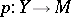,, was inspired by a paper by Ch. Ehresmann, [a1], where he analyzed the classical approaches to connections from the global point of view (cf. also Connections on a manifold; Fibre space; Manifold). The main idea is that at each point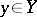one prescribes an-dimensional linear subspace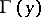of the tangent spaceofwhich is complementary to the tangent space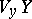of the fibre passing through. These spaces are called the horizontal spaces of. Henceis an-dimensional distribution on.

There are three main ways to interpret an Ehresmann connection:

1) As the lifting mapping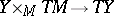, transforming every vector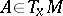into the unique vector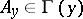satisfying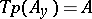,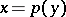. So, every vector fieldonis lifted into a vector field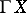on. The parallel transport onalong a curveonis determined by the integral curves of the lifts of the tangent vectors of.

2) As the connection form, transforming every vector of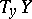into its first component with respect to the direct sum decomposition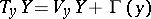. Since the vertical tangent bundleis a subbundle of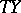, the connection form is a special tangent-valued one-form on.

3)is identified with an element of the first jet prolongation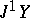of. Thenis interpreted as a section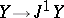.

Ifis a vector bundle and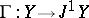is a linear morphism, thenis called a linear connection. (From this viewpoint, an Ehresmannn connection is also said to be a non-linear connection.) A classical connection on a manifoldcorresponds to a linear connection on the tangent bundle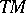. Ifis a principal fibre bundle with structure group, andis-invariant, thenis called a principal connection. These connections have been used most frequently. On the other hand, a big advantage of connections without any additional structure is that prolongation procedures of functorial character can be applied to them with no restriction.

The main geometric object determined byis its curvature. This is a section, whose definition varies according to the above three cases.

1) This is the obstructionfor lifting the bracket of vector fields,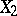on.

2)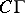is one half of the Frölicher–Nijenhuis bracket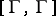of the tangent-valued one-formwith itself.

3) Consider the jet prolongation. Thencharacterizes the deviation of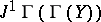from the second jet prolongationof, which is a subspace of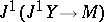.

The curvature ofvanishes if and only if the distributionis a foliation.

Every Ehresmann connection satisfies the Bianchi identity. In the second approach, this is the relation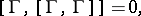which is one of the basic properties of the Frölicher–Nijenhuis bracket. For a classical connection on, this relation coincides with the second Bianchi identity.

For every section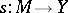, one defines its absolute differentialas the projection of the tangent mapping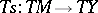in the direction of the horizontal spaces. Iterated absolute differentiation is based on the fact that every Ehresmann connection oninduces canonically an Ehresmann connection on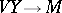, [a2].

If a tangent-valued one-formonis given, then the Frölicher–Nijenhuis bracketis called the-torsion of. This leads to a far-reaching generalization of the concept of torsion of a classical connection, [a3]. Even in this case, the basic properties of the Frölicher–Nijenhuis bracket yield a relation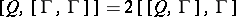which generalizes the first Bianchi identity of a classical connection.

A systematic presentation of the theory of Ehresmann connections (under the name of general connections) can be found in [a2].

How to Cite This Entry:
Ehresmann connection. Encyclopedia of Mathematics. URL: http://encyclopediaofmath.org/index.php?title=Ehresmann_connection&oldid=18987
This article was adapted from an original article by Ivan KolÃ¡Å™ (originator), which appeared in Encyclopedia of Mathematics - ISBN 1402006098. See original article